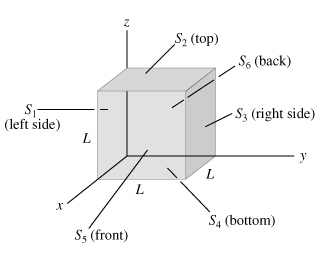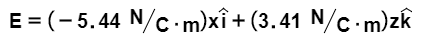# Problem: A cube has sides of length L = 0.330 m. It is placed with one corner at the origin as shown in the figure (Figure 1). The electric field is not uniform but is given byFind the electric flux through each of the six cube faces S1, S2, S3, S4, S5, and S6.Find the total electric charge inside the cube.

###### FREE Expert Solution

Electric flux is given by:

$\overline{){\mathbf{\varphi }}{\mathbf{=}}\stackrel{\mathbf{⇀}}{\mathbf{E}}{\mathbf{·}}{\stackrel{\mathbf{^}}{\mathbf{n}}}_{{\mathbf{s}}}{\mathbf{·}}{\mathbf{A}}}$

96% (56 ratings)###### Problem DetailsA cube has sides of length L = 0.330 m. It is placed with one corner at the origin as shown in the figure (Figure 1). The electric field is not uniform but is given byFind the electric flux through each of the six cube faces S1, S2, S3, S4, S5, and S6.

Find the total electric charge inside the cube.